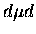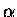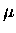Next: Direct stop contribution at Up: The Previous: Nitrogen contamination

##fusion

Protons fromfusion in the deuterium layer can cause a background in themeasurements depending on the energy cut (recall that the proton energy is 3 MeV). They can come from two different sources: (a) direct stopping of muons in the deuterium layer, or (b) recycled muons, i.e., the muons released after the fusion reaction (with the probability , whereis the sticking probability). For both cases, because of solid state effects and finite thickness, estimating the proton yield is difficult, and there is yet no satisfactory theoretical model. The use of the Monte Carlo is untested in these conditions, and its reliability is questionable without solid state effects included in the input.

Nonetheless, we first make an analytical estimate using the two node kinetics model which successfully, if accidentally, described the time evolution offusion in a bulk solid deuterium . According to this kinetics approximation, with an assumption of an infinite target and ignoringcycling,fusion yield per muon can be obtained as:

 = (108)

where is the target density in units of liquid hydrogen atomic density, are the initial hyperfine populations, ands-1 is the free muon decay rate. Using the effective formation rates from our measurements in thick solid deuterium targets [199,166], i.e., molecular formation rates from each hyperfine state,s-1,s-1, and the spin flip rate,s, we obtain . The fusion branching ratio into protons is a somewhat complicated function of atomic hyperfine states and molecular angular momentum states, but for our analysis here, it suffices to let . Hence we have the proton yield per muon in the kinetics model,

 (109)

of which 30% comes from the fast (34s-1) component, and the rest with the slower time slope.

This level of proton yield would give a non-negligible contribution in theyield measurements, when the direct stops and the recycling components are combined. However, a proton yield at the 10% level relative tocan be excluded by comparing the expected peak shape, simulated with an energy loss Monte Carlo program , with experimental energy spectra. Recall that the dd proton, with an energy of 3 MeV, has energy loss much smaller than a 3.5 MeV, by a factor of roughly , hence a 10% proton yield would appear as a sharp peak in the Si energy spectra, which is absent from the data. Given the inconsistency with this simple analytical approach, we pay closer attention to our data set to extract the proton contribution in the sections which follow.Next: Direct stop contribution at Up: The Previous: Nitrogen contamination